# August 2018 Puzzle Periodical - Crop Calculations

By Dr. James M., Operations Researcher

## Groups of Ring Fields

Creator Challenge Difficulty Rating: Medium

### Problem

A farmer, known for his unusual aesthetics, planted five circular fields. Four of the circles have a one furlong radius and were arranged so their centers form a square and they are each tangent to two of the other three circles. The fifth field was planted in the area enclosed by the four circles such that it is tangent to all of them.

What is the total area of the unplanted land enclosed by the five circles?

In this image, the fields are shown in green. We are interested in measuring the red regions.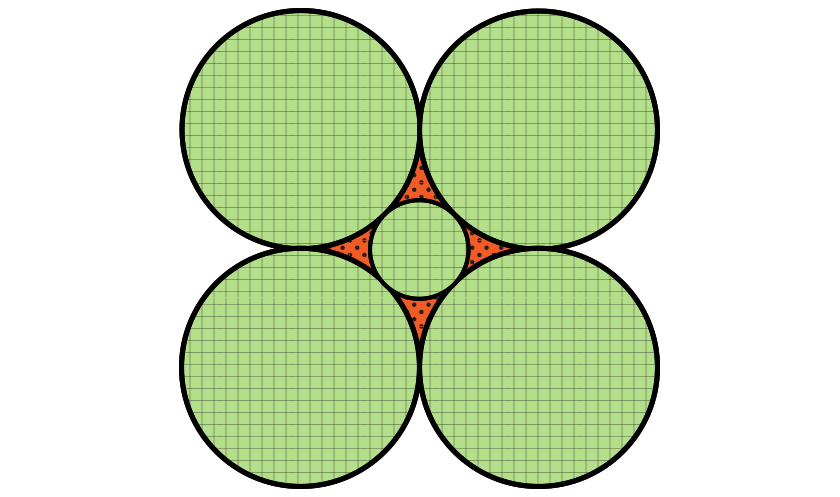### Solution

Did you calculate the amount of unplanted land enclosed by the five circles?

Our first step is to find the area bounded by the four larger circles. We can then subtract the area of the smaller circle to find the area we are interested in. Letbe the radius of the large circles. We know thatis equal to one furlong.

The square formed from the centers of the four larger circles has a side length of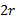. Thus it has an area of.If we exclude the region between the four larger circles, we see that we are left with four sectors, each containing a fourth of the area of its respective circle. The area of these regions is then.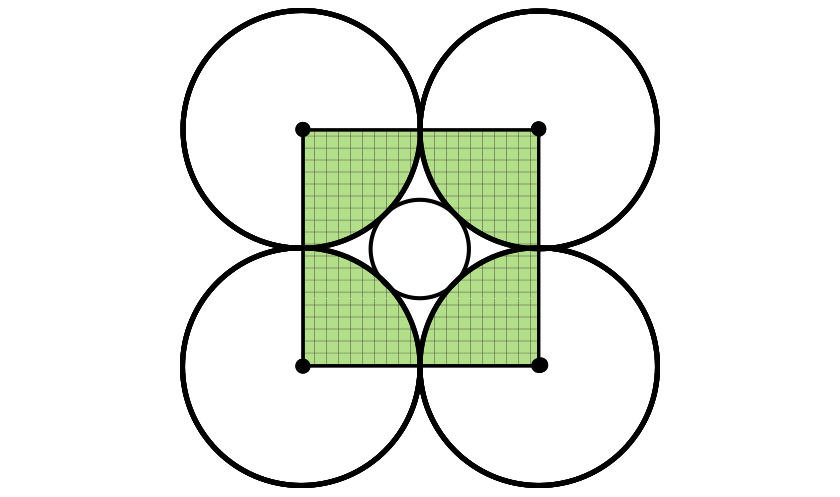Altogether then, the area of the region between the four larger circles is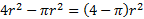.

Now we need to find the area of the smaller circle. The centers of any three of the larger circles form a 45-45-90 triangle whose hypotenuse is the diagonal of the square measured earlier. Its length is thus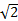times the side length of the triangle, which we know from before is. Thus the length of the diagonal is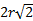. If we subtract the lengths of the two radii from this, we get the length of the diameter of the smaller circle. This length is thus. To get the radius of the smaller circle, we divide this by two, which gives us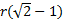. The area of the smaller circle is then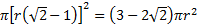.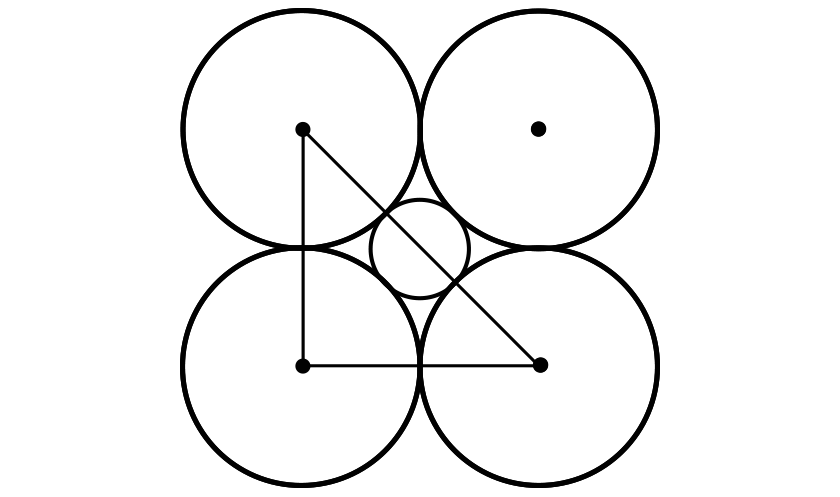We then get the area of the interior region by subtracting these two values, which is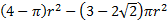. Plugging in the value of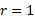furlong gives us the final answer of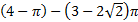square furlongs, which is about .32 square furlongs.